- DSP log - http://www.dsplog.com -

Posted By Krishna Sankar On June 16, 2009 @ 4:26 am In Modulation | 47 Comments

In a post on Minimum Shift Keying (MSK) , we had discussed that MSK uses two frequencies which are separated by and phase discontinuity is avoided in symbol boundaries. In that post, we had discussed MSK as a continuous phase transmit signal and showed that phase changes through 0, 90, 180 and 270 degrees. In this post, we will discuss MSK transmission as a variant of offset-QPSK technique. Further, we will discuss the receiver structure and show that bit error rate with coherent demodulation of MSK (using time) is equivalent to that of BPSK modulation. The channel assumed is AWGN.

## MSK transmitter

Using bit-sequence and the explanation provided in the excellent paper  Minimum shift keying: A spectrally efficient modulation  Subbarayan Pasupathy, IEEE Communications Magazine, July 1979 as a reference,  let us proceed as follows:

a) Consider a 8 bit sequence which is BPSK modulated as [+1, +1, -1, -1, -1, +1, +1 , +1] having symbol duration of .

b) The same sequence can be sent over QPSK modulation, where even bits are send on I-arm and the odd bits are send over Q-arm. To keep the same data rate, the bits on the I/Q arm are send for symbol periods.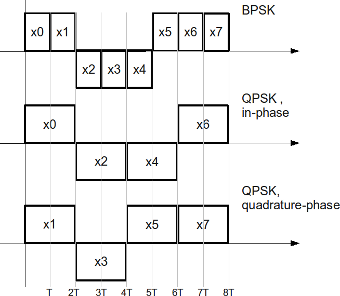Figure: Transmission of BPSK/QPSK modulation

c) Now, a variant of QPSK called offset-QPSK (O-QPSK)  can be generated by having a relative delay between the I-arm and Q-arm by symbol period .

Note: The advantage of O-QPSK is that phase of the signal can jump at a maximum of only 90 degrees (when compared to 180 degrees in QPSK). Having a smaller phase jump ensures that the spectrum of the waveform is cleaner even when there are distortions in the transmitter.

d) Researchers have found that they can make the phase transitions zero, if  rectangular pulse shapes used in O-QPSK are replaced by sinusoidal pulse shapes i.e by using and on the I and Q arm respectively. This is also known as Minimum Shift Keying (MSK) !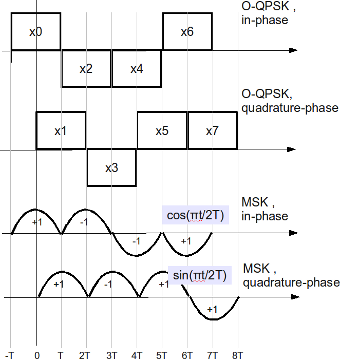Figure: Transmission of O-QPSK/MSK modulation

Assuming that the carrier frequency is , the MSK transmission can be written as

,

where

are the even pulse sequence (send on the I arm) and

are the odd pulse sequence (send on the Q arm).

Using trigonometric identities, the above equation can also be written as,

,

where

and

.

We can see that MSK can indeed be visualized as a form of frequency shift keying (FSK), where the two frequencies are and .

Note: This equation is comparable to that described in the post Simulating MSK transmission 

The MSK transmitter block diagram is shown below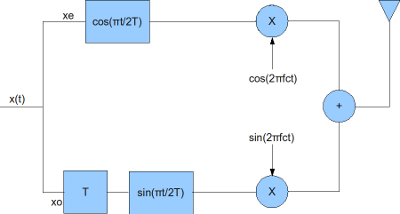Figure: Block diagram of MSK transmitter

The receiver for the MSK transmission can be constructed as follows:

a) Down convert the RF signal to I and Q arms by I/Q down conversion

b) Multiply the I and Q arms by and respectively

c) Integrate over the a period of

d) On I arm, perform hard decision decoding on the integrator output at every to get the even bits

e) On Q arm, delay by time , perform hard decision decoding on the integrator output at every to get the odd bits

The block diagram is shown below: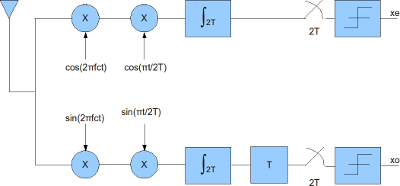## Simulation Model

The Matlab/Octave script performs the following

(a) Generate random binary sequence of +1’s and -1’s.

(b) Group them into even and odd symbols

(c) Perform rectangular pulse shaping on the even and odd symbols, delay the odd symbols by

(d) Multiply the even and odd symbols by and respectively and transmit

(e) Add additive white Gaussian noise (AWGN) for the given value of

(f) Multiply the I and Q arm by  and respectively and integrate over period

(g) Using the I arm, recover even bits by performing hard decision decoding on integrator output every time

(h) Using the Q arm, recover even bits by performing hard decision decoding on integrator output delayed by time every time

(i) Count the bit errors

(j) Repeat for multiple values of and plot the simulation and theoretical results.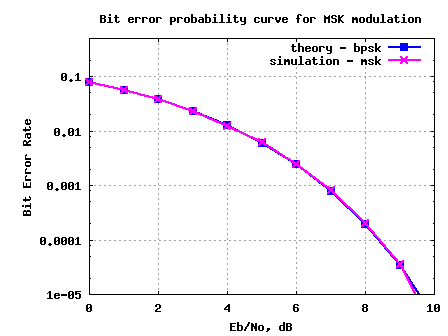Figure: BER plot for MSK transmission/reception in AWGN channel

## Observations

1./ The BER with MSK is identical to BER with BPSK modulation . This is because MSK demodulation in the above simulation is performed by integrating the received symbol over  time .

2./ Note that it is indeed possible to demodulate MSK by observing only over time and demodulate it as a FSK with two carriers at and . If such a demodulation is performed, then the BER with MSK will be 3dB poorer (comparable to FSK demodulation ) when compared to BPSK modulation.

## Reference

Minimum shift keying: A spectrally efficient modulation  Subbarayan Pasupathy, IEEE Communications Magazine, July 1979

URLs in this post:

 Minimum Shift Keying (MSK): http://www.dsplog.com/2008/01/19/simulating-minimum-shift-keying-transmitter/

 Minimum shift keying: A spectrally efficient modulation: http://ieeexplore.ieee.org/stamp/stamp.jsp?arnumber=01089999

 offset-QPSK (O-QPSK): http://en.wikipedia.org/wiki/QPSK#Offset_QPSK_.28OQPSK.29

 Matlab/Octave script for computing BER with MSK transmission and reception in AWGN channel: http://www.dsplog.com/db-install/wp-content/uploads/2009/06/script_ber_msk_awgn.m

 BER with BPSK modulation: http://www.dsplog.com/2007/08/05/bit-error-probability-for-bpsk-modulation/

 FSK demodulation: http://www.dsplog.com/2007/08/30/bit-error-rate-for-frequency-shift-keying-with-coherent-demodulation/